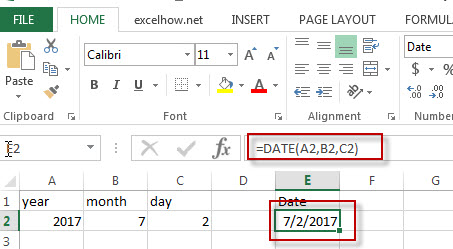# Excel Date Function

This post will guide you how to use Excel DATE function with syntax and examples in Microsoft excel.

### Description

The Excel DATE function returns the serial number for a date.

The DATE function is a build-in function in Microsoft Excel and it is categorized as a DATE and TIME Function.

The DATE function is available in Excel 2016, Excel 2013, Excel 2010, Excel 2007, Excel 2003, Excel XP, Excel 2000, Excel 2011 for Mac.

### Syntax

The syntax of the DATE function is as below:

```= DATE (year, month, day)
```

Note:

• If the year value is between 0 and 1899, excel will add that value to 1900 to determine the year.
• If the year value is between 1900 and 9999, excel will use that value as the year value.
• If year is less than 0 or is greater than 9999, excel will return the error value: #NUM!
• If month is greater than 12, every 12 months will add 1 year to the year value.
• If the day value is greater than the number of days in the month specified, then it will add that number of days to the first day in the month. For example: DATA(2017,6,32), the DATE function will return “7/2/2017”

### Example

The below examples will show you how to use Excel DATE Function to return the sequential serial number for a date.

#1 =DATE(A2,B2,C2)### More Excel DATE Function Examples

• Calculate Thanksgiving Date
You can use a simple arithmetic to calculate the possible date range for the US Thanksgiving, it falls on the fourth Thursday in November. And you can create a formula based on the DATE function and the WEEKDAY function.…
• Get the First Monday of a Given Year
To calculate the first Monday of the year or given any date, you can create a new complex formula based on the DATE function, the YEAR function and the WEEKDAY function……
• Convert Month Name to Number
If you want to convert month name to number with an excel formula, or you want to convert 3 letter month name to numbers, you can create a formual based on the MONTH function and the DATEVALUE function…
• Converting Week Number to Date
You need to create a formula based on the MAX function, the DATE function and the WEEKDAY function to convert week number to a date in Excel.…